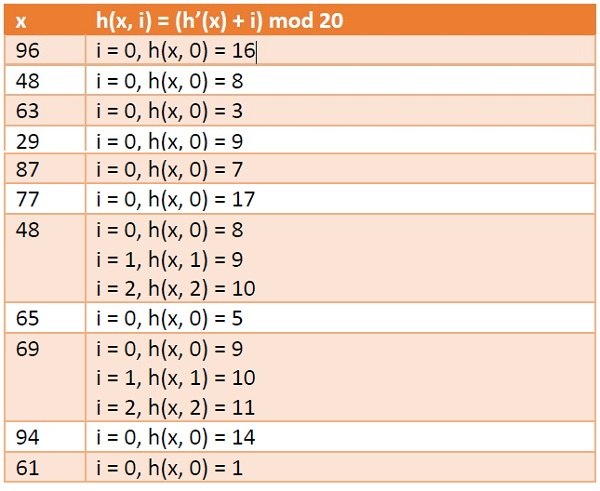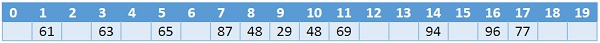# Linear Probing in Data Structure

In this section we will see what is linear probing technique in open addressing scheme. There is an ordinary hash function h´(x) : U → {0, 1, . . ., m – 1}. In open addressing scheme, the actual hash function h(x) is taking the ordinary hash function h’(x) and attach some another part with it to make one linear equation.

h´(𝑥) = 𝑥 𝑚𝑜𝑑 𝑚

ℎ(𝑥, 𝑖) = (ℎ´(𝑥) + 𝑖)𝑚𝑜𝑑 𝑚

The value of i| = 0, 1, . . ., m – 1. So we start from i = 0, and increase this until we get one freespace. So initially when i = 0, then the h(x, i) is same as h´(x).

## Example

Suppose we have a list of size 20 (m = 20). We want to put some elements in linear probing fashion. The elements are {96, 48, 63, 29, 87, 77, 48, 65, 69, 94, 61}Hash TableUpdated on: 10-Aug-2020

5K+ Views